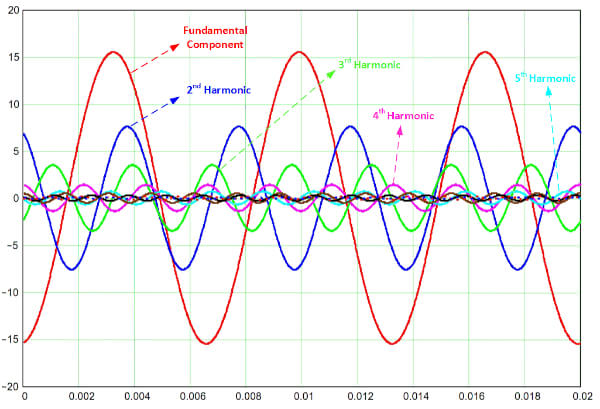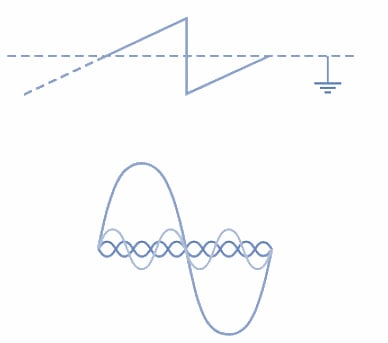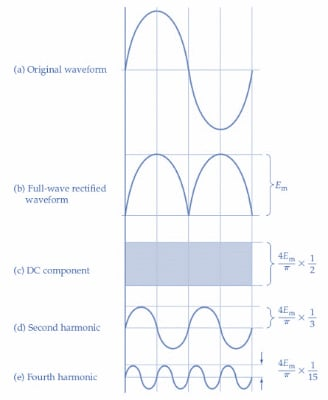Technical Article

# Harmonics Analysis: Using Fourier to Analyze Waveforms

August 15, 2022 by Amna Ahmad

## All repetitive waveforms can be composed of combinations of many sinusoidal waves. Any waveform can be analyzed to determine the component quantities. In this article, learn how to use Fourier Analysis to determine the amplitudes of harmonic components and their phase relationship to the fundamental component in various periodic non-sinusoidal waveforms.

A harmonic is a frequency that is an integer (whole number) multiple (second, third, fourth, etc.) of the fundamental frequency. The fundamental frequency on power distribution lines is 60 Hz and changes from positive to negative 60 cycles per second. For instance, the second harmonic on a 60 Hz power distribution line is 120 (60 × 2) Hz. The second harmonic waveform completes two cycles during one cycle of the fundamental waveform over the same period of time.##### Figure 1. A harmonic is a frequency that is an integer (whole number) multiple (second, third, fourth, fifth, etc.) of the fundamental frequency. Image used courtesy of Amna Ahmad

Fourier analysis (developed by mathematician Jean Fourier) is a mathematical operation that analyzes the waveforms to determine their harmonic content. Each harmonic's amplitude, as well as its phase relationship to the fundamental, can be determined. Also, the level of any DC component can be computed.

### Square Wave

A pure square wave, symmetrical above and below ground level [Figure 2], can be shown by Fourier analysis to be represented by the following equation:

$e=\frac{4E_{m}}{\pi}{\LARGE[}sin\omega t+\frac{sin3\omega t}{3}+\frac{sin5\omega t}{5}+\frac{sin7\omega t}{7}+...{\LARGE]}(1)$

where

e is an instantaneous value at time t

$$\frac{4E_{m}}{\pi}$$ is the peak value of a waveform

sinωt is a Fundamental component

$$\frac{sin3\omega t}{3}$$ is a Third harmonic

$$\frac{sin5\omega t}{5}$$ is a Fifth harmonic

$$\frac{sin7\omega t}{7}$$ is a Seventh harmonic

As shown by the equation, a symmetrical square wave can be made up of fundamental component and odd harmonics but has no even harmonics and no DC component.##### Figure 2. Harmonic analysis of a symmetrical square wave shows that it contains fundamental and odd harmonics. Image used courtesy of Amna Ahmad

NOTE: Fourier analysis can be applied to all repetitive waveforms to determine their harmonic content.

### Sawtooth Wave

The Fourier equation for the sawtooth waveform in Figure 3 is

$e=\frac{2E_{m}}{\pi}{\LARGE[}sin\omega t-\frac{sin2\omega t}{2}+\frac{sin3\omega t}{3}-\frac{sin4\omega t}{4}+\frac{sin5\omega t}{5}...{\LARGE]}(2)$

In this case, all the harmonics are present, and again, there is no DC component. In general, a waveform has no DC component when it is symmetrical above and below ground level.### Rectified Wave

The full-wave rectified sine wave in Figure 4 can be represented by

$e=\frac{4E_{m}}{\pi}{\LARGE[}\frac{1}{2}+\frac{cos2\omega t}{3}-\frac{cos4\omega t}{15}+\frac{cos6\omega t}{35}...{\LARGE]}(3)$##### Figure 4. A full-wave rectified sine wave comprises a DC component and even harmonics that decrease in amplitude with increasing harmonic number. Image used courtesy of Amna Ahmad

Equation 3 shows that the waveform has a DC component $$\frac{4E_{m}}{2\pi}$$ and even harmonics, 2ωt, 4ωt, 6ωt, and so on ( Figure 4). It would appear there is no fundamental frequency component. However, in this case, the fundamental frequency is taken as the input frequency (f) of the waveform prior to rectification. It could be argued that the fundamental frequency of the rectified waveform is actually 2f. For example, a 60 Hz sine wave, when full-wave rectified, produces a succession of sinusoidal half-cycles with a frequency of 120 Hz.

### Amplitudes of Harmonics

Examination of the equations for the square, sawtooth, and rectified sine wave shows that in all cases, the amplitudes of the harmonic components decrease as the harmonic frequency increases. Thus, the higher-order harmonics appear to have decreasing importance. This is certainly true in terms of the contribution of these components to the rms value of the waveform and to the power dissipated in a load. However, for good reproduction of the waveform, many of the higher-order harmonics must be present. For example, in the case of a square wave, all components up to the eleventh harmonic (or higher) may be required. For a pulse waveform, harmonics up to the one hundredth may have to be present to create a good output wave shape.

### Square Wave Example

A square wave with a 2 V peak-to-peak amplitude is symmetrical above and below ground level. Calculate the amplitudes of each component up to the seventh harmonic.

Solution.

From equation (1),

$e=\frac{4E_{m}}{\pi}{\LARGE[}sin\omega t+\frac{sin3\omega t}{3}+\frac{sin5\omega t}{5}+\frac{sin7\omega t}{7}+...{\LARGE]}$

Em = 1 V (Peak)

$\frac{4E_{m}}{\pi}=\frac{4\times1}{\pi}=1.27V$

Fundamental                    $$E_{m1}=\frac{4E_{m}}{\pi}=1.27V$$

Third Harmonic                    $$E_{m3}=\frac{4E_{m}}{3\pi}=0.42V$$

Fifth Harmonic                    $$E_{m5}=\frac{4E_{m}}{5\pi}=0.52V$$

Seventh Harmonic                    $$E_{m7}=\frac{4E_{m}}{7\pi}=0.18V$$

Note that the harmonic voltage components calculated are all peak values. Each must be multiplied by 0.707 to determine the rms values.

### Rectified Sine Wave Example

A full-wave rectified sine wave has a peak amplitude of 30 V and a (pre-rectified) frequency of 60 Hz. Calculate the DC component and the rms values of the harmonic components up to the sixth harmonic. Also, determine the harmonic frequencies.

Solution.

From equation (3),

$e=\frac{4E_{m}}{\pi}{\LARGE[}\frac{1}{2}+\frac{cos2\omega t}{3}-\frac{cos4\omega t}{15}+\frac{cos6\omega t}{35}...{\LARGE]}$

$\frac{4E_{m}}{\pi}=\frac{4\times30}{\pi}=38.2V$

DC Component   $$E_{dc}=\frac{4E_{m}}{\pi\times2}=19.1V$$

Fundamental   $$E_{m1}=0$$

Second Harmonic                    $$E_{m2}=\frac{4E_{m}}{3\pi}=12.7V$$
$$E_{2}=0.707\times E_{m2}=9V$$
$$f_{2}=2f_{1}=2\times60=120 Hz$$

Fourth Harmonic                    $$E_{m4}=\frac{4E_{m}}{15\pi}=2.5V$$
$$E_{4}=0.707\times E_{m4}=1.8V$$
$$f_{4}=4f_{1}=4\times60=240Hz$$

Sixth Harmonic                    $$E_{m6}=\frac{4E_{m}}{35\pi}=1.1V$$
$$E_{6}=0.707\times E_{m6}=0.78V$$
$$f_{6}=6f_{1}=6\times60=360Hz$$

### Conclusion

With harmonic analysis, periodic non-sinusoidal waveforms can be shown to consist of combinations of pure sine waves, sometimes with a DC component. One main component, a large-amplitude sine wave having the same frequency as the periodic wave being analyzed, is the fundamental. The other components are sine waves with frequencies that are exact multiples of the fundamental frequency. These waves, denoted as harmonics, are numbered according to the ratio of their frequency to that of the fundamental.

Featured image used courtesy of Adobe Stock